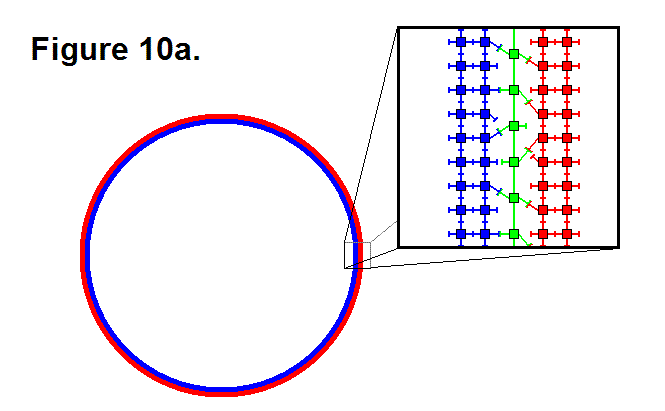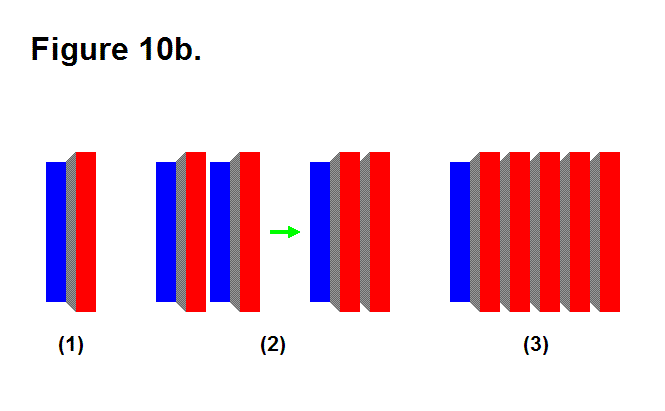# MATRIX PRODUCTION OF ROTATIONAL MOTION

A matrix consisting of a large quantity of modules is capable of producing rotational motion. Figure 10a, shown below, shows a matrix in a ring configuration to produce this effect. It consists of an outer ring in red, and an inner ring in blue. It can be more than one ring layer of modules thick. The box shows an enlarged “under the microscope” view of the individual modules that make up a portion of the ring matrix. The center column, in green, consists of modules that repeatedly connect and disconnect to the red and blue columns of modules and forms a ring layer in the matrix between the red and blue rings. For the red ring to produce a clockwise rotation motion in the blue ring, the legs on the modules in the green column and on the modules in the columns on either side of the green column that connect to the green column pivot clockwise when not connected together and counter-clockwise when connected together. They do the reverse to produce a counter-clockwise rotational motion in the red ring.The maximum rotational speed produced by this matrix structure is fairly slow; for example, suppose that it has a radius of 10 feet; it will hypothetically only be able to produce 1 cycle per minute of rotational motion (or 1 RPM). By connecting several matrices of rings together in series in a certain way the angular speed can be doubled for each additional layer. Figure 10b, shown below, shows a matrix in image (1) consisting of two cylinders in red and blue connected together by a truncated cone section in gray. This matrix structure by itself does not produce rotational motion; it is a rigid object. Image (2) shows how two matrix objects structured this way can combine to form another complex matrix structure that layers the blue section for the matrix on the right in the red section of the matrix on the left to produce rotational motion, which corresponds to the matrix in Figure 10a. The five matrices connected together in series in image (3) have 4 layers that can produce a relative rotational speed that is 8 times the rotational speed of a single layer of 2 matrices connected together between the matrix structures at the ends of this complex matrix structure. The calculation is 25-2 = 8, where the 5 is the number of matrix structures and the rest of the numbers are constants. If 1 layer is capable of producing a maximum of 1 rotation per minute, then 4 layers is capable of producing 8 rotations per minute and 12 layers is capable of producing somewhere in the vicinity of two thousand rotations per minute (hypothetically, 2,048 RPM).In general, the hypothetical formula for the total maximum rotational speed output is

sn-1 = 2n-2∙s1

where sn-1 is the total maximum rotational speed, n is the number (greater than 1) of matrix structure objects such as the one in image (1) of Figure 10b, and s1 is the maximum rotational speed output of two of these matrix structure objects. This means a very large rotational speed can be produced by having many matrices that produce rotational motion in series, but this is limited by the tension produced by the centrifugal forces in the matrices that are rotating at faster speeds. Factors such as radius, density of the modules (mass), friction from wind resistance, and maximum tension that the modules can handle have to be taken into consideration to determine the actual upper limit of sn.